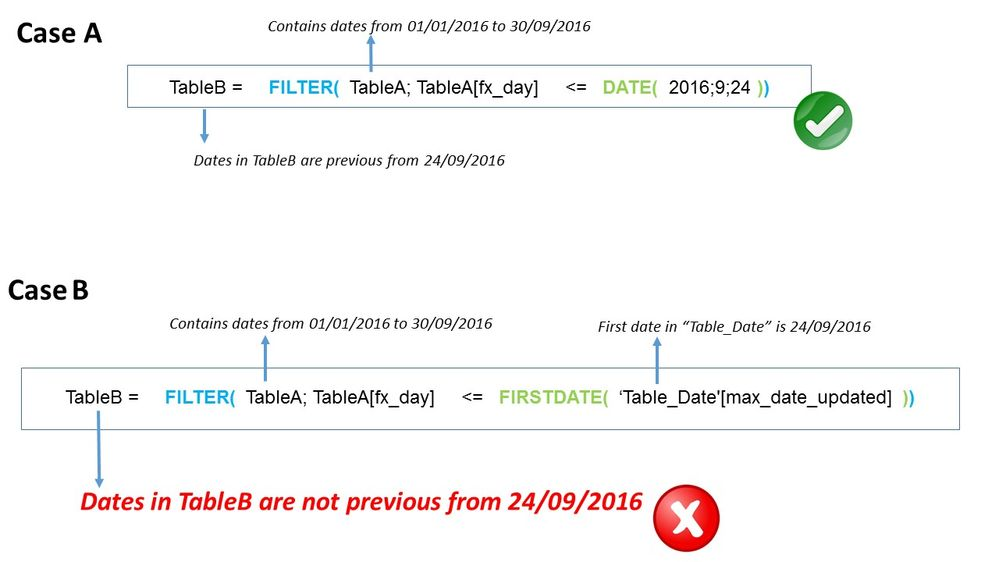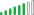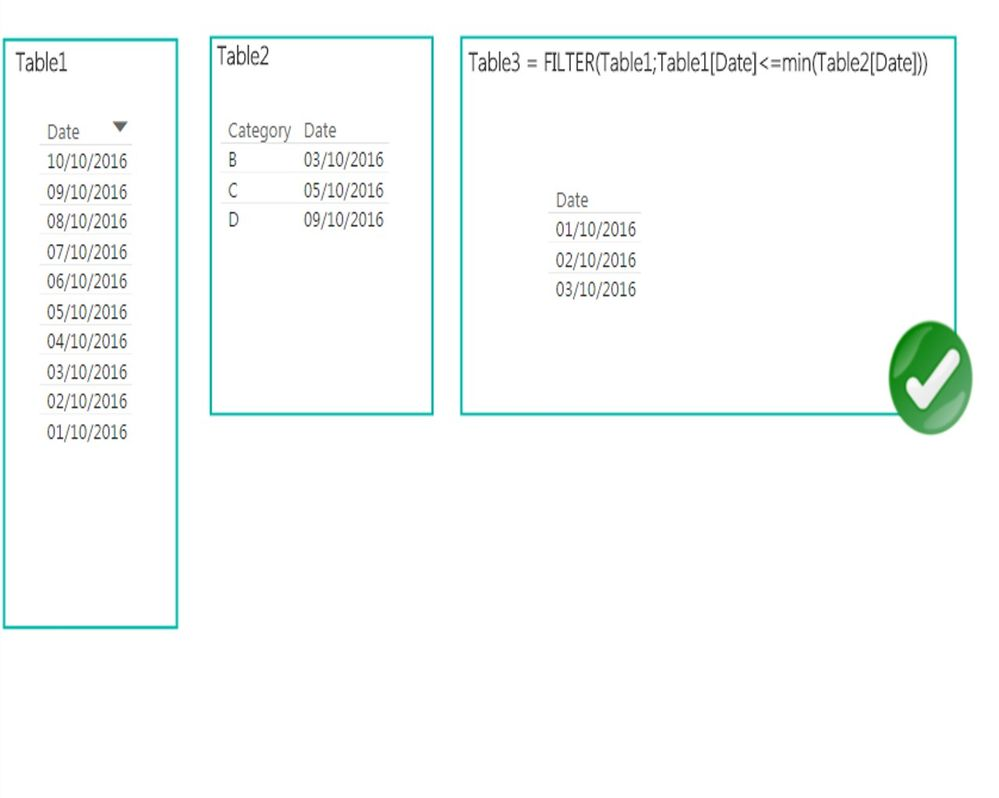cancel
Showing results for
Did you mean:Regular Visitor

## Does function FILTER() work with another function given as a parameter?

Hi,

I’m trying to calculate table B from filtered table A (the filter condition is a cutting date):Does anyone know what I'm doing wrong in case B?

1 ACCEPTED SOLUTIONSolution Supplier

In your file Table3 is a calculated table ...

Calculated tables are static parts of a model : their content will not change based on selections or slicers.

You can use table expressions in measures to obtain dynamic selections, though ...

6 REPLIES 6Solution Supplier

This may be related to: http://www.sqlbi.com/articles/usage-of-dates-argument-in-a-row-context/

The short explanation is: FIRSTDATE has an implicit CALCULATE and is evaluated depending on the row context of TableA.

If correct, your condition probably evaluates to TRUE for each row.

Try with the MIN function instead.Regular Visitor

Hi Laurent Cuarto, first of all many thanks for your prompt response.

I would like to apologise for don't explain correctly the situation before.

You can see in Case C the real problem. In fact, once I give the measure to the filter function,  the filter doesn't work as I want.It seems that the filter function doesn't accept a measureSolution Supplier

The article I linked to explains this in details.

For the short explanation ...

`FILTER( Table, Table[date] <= FIRSTDATE(Dates[date]) )`
`FILTER( Table, Table[date] <= CALCULATE(MIN(Dates[date])) ) `
`FILTER( Table, Table[date] <= [MINDATE measure] )`

... will all give you unexpected results because the right-hand side of your filter expression is calculated within the row context (it returns the date for the current row, that is Table[date]).

As a consequence, your inequality always evaluates to true.

On the contrary, this should work:

`FILTER( Table, Table[date] <= MIN(Dates[date]) )`

The reason is this MIN(Dates[date]) is calculated for the current filter context your measure is evaluated in returns the same result for all rows in Table. As a consequence, it will be true for some rows and not for others.Regular Visitor

Hi LaurentCuarto,

I would like to apologise, I haven't explained well what I'm trying to do.

I've created this example in order to explain it better.

Case A:Case B:In these examples, Table 1 and Table 2 are input data.

In case A the result (Table 3), is filtered according to the minimum of Table 2[Date], that is correct.

In case B the result (Table 3), isn't filtered according to the minimum of the filter (slicer), that references Table 2. In case B, the minimun date in the filter (slicer) is 05/10/2016, but Table 3 is cut by the date 03/10/2016, what isn't correct, it should be cut by the date 05/10/2016,

I attach the link where is the Power BI Desktop document, that contains this example, just in case you want to open it.

https://www.dropbox.com/s/7tawmtjz8a1ytdz/ej1.pbix?dl=0

CheersSolution Supplier

In your file Table3 is a calculated table ...

Calculated tables are static parts of a model : their content will not change based on selections or slicers.

You can use table expressions in measures to obtain dynamic selections, though ...Regular Visitor

Actually I don't need to calculate any table, so I can get by with thise:

SUMX(

FILTER(table1;table1[date]<=MIN(dates_min[date]))

;table1[A])

Thank you very much

CheersAnnouncements#### Exclusive opportunity for Women!

Join us for a free, hands-on Microsoft workshop led by women trainers for women where you will learn how to build a Dashboard in a Day!#### Power Platform Conference-Power BI and Fabric Sessions

Join us Oct 1 - 6 in Las Vegas for the Microsoft Power Platform Conference.Top Solution Authors
Top Kudoed Authors
Users online (1,721)